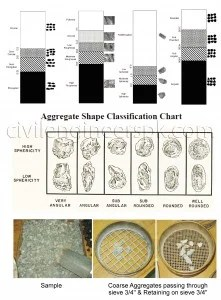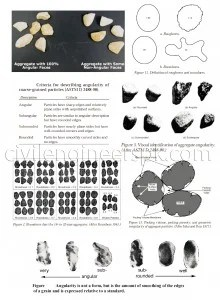# Exp 2 Aggregate Angularity Number

## EXPERIMENT NO. 2

### Determination of Angularity Number for the given aggregate sample.

This test is also carried out for determining shape of the aggregates. Based upon shape the aggregates may be classified as Rounded, Angular or Flaky. Angular particles possess well defined edges formed at the intersection of roughly planer faces and are commonly formed in aggregates prepared by crushing of rocks.

Angularity in general is the absence of rounding of particles of an aggregate. This test is performed to determine the angularity number i.e. the absence of roundedness or the degree of angularity of the aggregate specimen.

RELATEDTHEORY

SHAPES OF PARTICLES

The usual shapes of the particles are;

1. Rounded (river gravel)
2. Flaky (laminated rock)
3. Elongated
4. Angular (crushed rock)

ANGULARITY

It is the absence of roundness. An aggregate particle, which is more rounded, is less angular and vice versa.

ANGULARITY NUMBER

Angularity number of an aggregate is the amount (to the higher whole number) by which the percentage of voids in it after compacting in a prescribed manner exceeds 33.

Where, “33” is the percentage of volume of voids, in a perfectly rounded aggregate. “67” is the percentage of volume of solids in a perfectly rounded aggregate.

The value of angularity number generally lies between 0 & 11. In road construction angularity number of 7 – 10 is generally preferred.

SIGNIFICANCE

•  The degree of packing of particles of single sized aggregate depends upon the angularity of aggregate.
• The angularity of the aggregate can be estimated from the properties of voids in a sample of aggregate compacted in a specified manner.
• The angularity number ranges from 0 for a highly rounded grave to about 11 for freshly crushed angular aggregates.
• Higher the angularity number, more angular and less workable is the concrete mix.
• In cement concrete roads (rigid pavements) rounded aggregates are preferred because of better workability and higher strength.
• In bituminous or water bound macadam construction (like flexible pavements), angular aggregates with high angularity number are preferred because of high stability due to better interlocking and friction.
• Higher the angularity number, more angular and less workable is the concrete mix.
• In road construction, angularity number of 7 -10 is generally preferred.

APPARATUS

1 –  A metal cylinder of about 3-liter capacity.2 –  Temping rod of circular cross-section, 16mm Φ, 60cm in length. Rounded at one end.

3 –  A metal scoop.

4 –  A weighing balance.

PROCEDURE

This procedure is for aggregate size ¾ to No.4. If aggregate is coarser than ¾, a cylinder of large capacity shall be required but amount of compactive effort or energy should be proportional to the volume of the cylinder.10 Kg of the sample is taken for the test. The material should be oven dried. The aggregate is compacted in three layers, each layer being given 100 blows using the standard tamping rod at a rate of 2 blows/second by lifting the rod 5 cm above the surface of the aggregate and then allowing it to fall freely. The blows are uniformly distributed over the surface of the aggregate. After compacting the third layer, the cylinder is filled to overflowing and excess material is removed off with temping rod as a straight edge.

The aggregate with cylinder is then weighed. Three separate determinations are made and mean weight of the aggregate in the cylinder is calculated.

## Calculations & Results

Method – 1Add measured quantity of water in the compacted aggregate till all the voids are filled and water appears to the surface. Volume of water added is approximately equal to the volume of voids in the compacted aggregate.

Angularity Number = (Volume of water added/Total volume ) x 100-33

Method – 2

Angularity Number = 67-(100W/CGs)

where,
W = mean weight of the aggregate filling cylinder.
C = Weight/Volume of water that can completely fill the cylinder (= 3 liters = 3000 ml – in our lab)
Gs = Specific Gravity of the aggregate.

Notes:

1-    Method – 1 determines the angularity number from the solids point of view.
2-    Method – 2 determines the angularity number from the voids point of view.

Observations & Calculations

Specific gravity of the aggregate = 2.67
Total volume of the cylinder = 3042.18  cm3

 Mean Value to be used Weight (grams) Volume of Water added (ml) Angularity Number Empty Cylinder Cylinder + Aggregates Cylinder + Aggregates + Water Aggregate Method  1 Method  2 5040 9948 11233 4931.67 1261.33 8 6 5040 10032 5040 9935 5040 9971.67

Specific gravity of the aggregate = 2.67
Total volume of the cylinder = 2970  cm3

 Mean Value to be used Weight (grams) Volume of Water added (ml) Angularity Number Empty Cylinder Cylinder + Aggregates Cylinder + Aggregates + Water Aggregate Method  1 Method  2 5040 9948 11233 4931.67 1261.33 9 5 5040 10032 5040 9935 5040 9971.67

In the first table we have used the volume written on the container but in the second container we poured water and then measured the volume , the container was not fully filled, So we can say the first table is more reliable and the difference between both the methods may be due to the face that we did not measure the specific gravity. We used 2.67 which can be different then the actual value but both the methods should give the same result.

Angularity Number, Angularity Number, Angularity Number, Angularity Number, Angularity Number, Angularity Number, Angularity Number

## 4 Replies to “Exp 2 Aggregate Angularity Number”

1. It’s very helpful.. thanks alot

•CIVILENGINEERSPK on said:

Welcome

•Wassim on said:

can you please provide the related standard?

•CIVILENGINEERSPK on said: